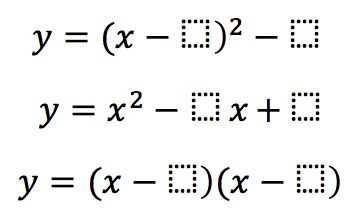Directions: Using the digits 1 to 9 at most one time each, place a digit in each box so that each quadratic is the same.### Hint

What form can you get each quadratic into? What happens to the numbers in the boxes when that happens?

Have you tried assigning a letter to any of the boxes?

Where letters, A,B,C,D,E,F are the boxes in order of appearance,
A=3
B=1
C=6
D=8
E=2
F=4
(E and F are interchangeable)

Source: John Rowe

## Period of Trig Function 3

Directions: Using the digits 0 to 9, at most one time, fill in the blanks …